# Pythagoras' theorem

By Martin McBride, 2023-01-09
Tags: triangle solving triangles
Categories: gcse trigonometry pythagorasThis chapter covers Pythagoras' theorem, its proof and its applications.

Here is a video covering this:

## Pythagoras' theorem

Pythagoras' theorem applies to right-angled triangles.

If we know any two sides of a right-angled triangle, Pythagoras' theorem allows us to calculate the third side.

This right-angled triangle has three sides, of length a, b and c: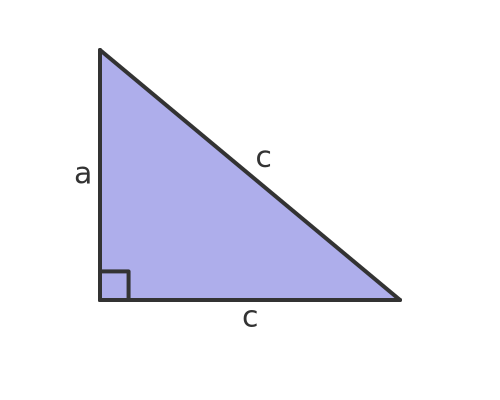This side opposite the right angle, side c, is called the hypotenuse. It is always the longest side.

The theorem tells us:

The square of the hypotenuse is equal to the sum of the squares of the two opposite sides.

This can be expressed as a formula: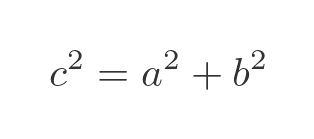Where c is the hypotenuse, a and b are the other two sides. This diagram shows the triangle with a square drawn on each side. The area of the square drawn on side c is equal to the sum of the areas of the other two squares: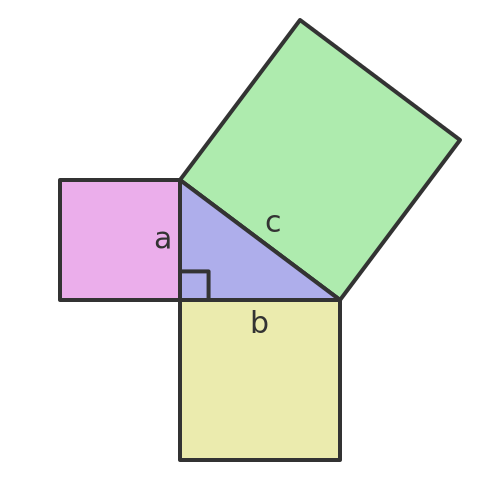## Alternative formulas

If we know a and b we can find c, using the previous formula:We can take the square root of both sides: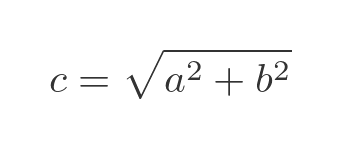If we know b and c we can find a, rearranging the original formula: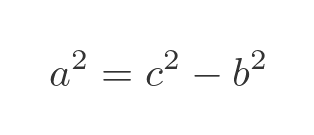We can take the square root of both sides: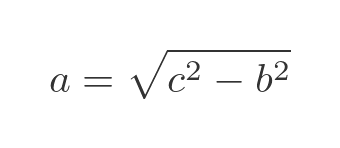This similar equation allows us to find b if we know a and c: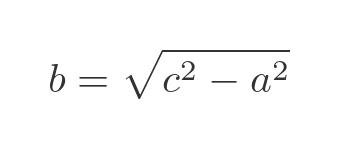## Example 1

Here is a triangle where we know that side a = 3 and b = 4: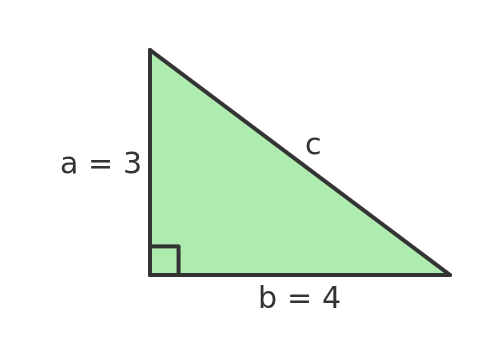If we use the formula above we can find c:Substituting for a and b: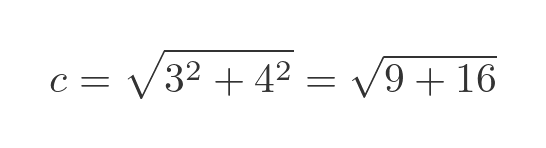This gives: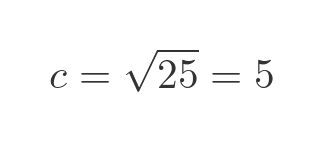In this case, c2 is 25, which is a perfect square, so the side c has a nice integer value of 5.

To see how this works, we can divide each of the squares into a grid of smaller squares, each of size 1 by 1.: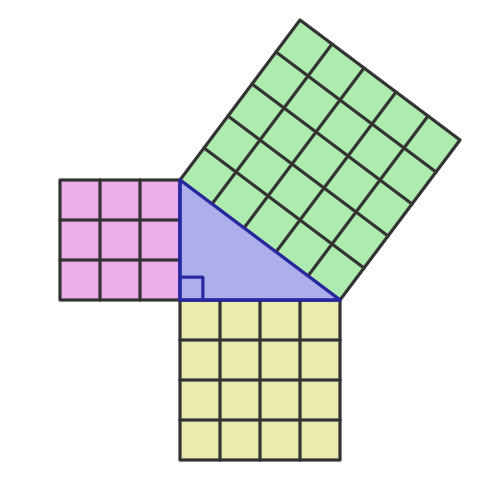The left-hand (magenta) square has side length 3, so it is made of 3 by 3 small squares. The lower (yellow) square has side length 4, so it is made up of 4 by 4 small squares. The green square, on the hypotenuse, has side length 5, so it is made up of 5 by 5 small squares.

Here is how the two small squares add up to the same area as the large square. If we take the 9 magenta squares and add them around the edge of the 16 yellow squares square, we get a 5 by 5 square: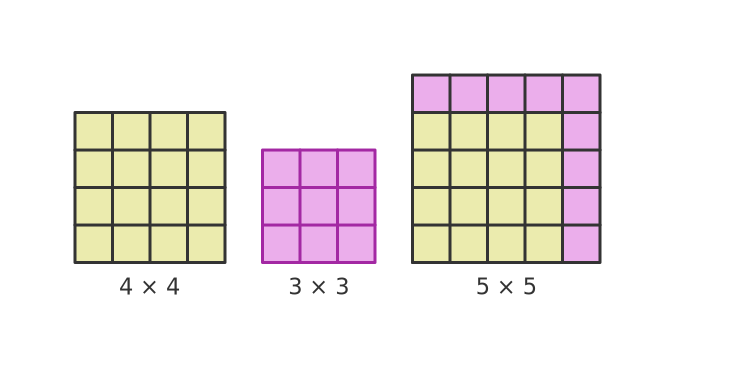A right-angled triangle where all three sides have integer lengths is a special case. This particular case is called a 3, 4, 5 triangle. We call the numbers (3, 4, 5) a Pythagorean triple.

There are other cases, for example, a right-angled triangle with sides 5 and 12 has a hypotenuse of length 13. So (5, 12, 13) is also a Pythagorean triple.

Most right-angled triangles do not have all sides of integer length. Even if two of the sides have integer lengths, the third will usually have a length that involves a square root term.

## Example 2

This time we will find side a when we know sides b and c.

Here is a triangle with b = 2 and c = 3: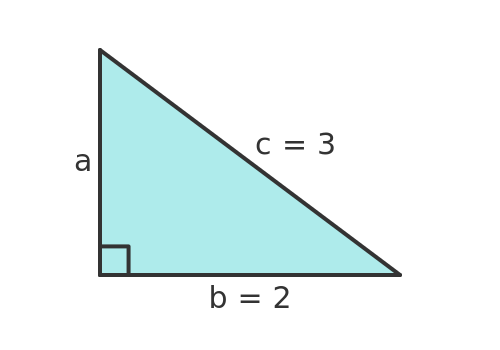If we use the formula above we can find c:Substituting for b and c: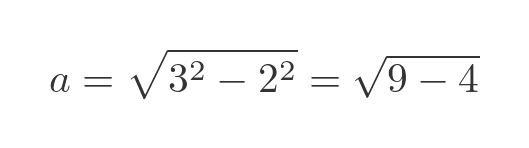This gives: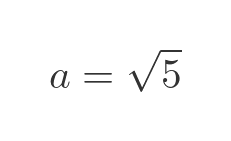In this case, a2 is 5. Since 5 is not a square number, we can't find an exact value of a. It is approximately 2.2361, but its exact value can only be expressed as a surd.

## Example 3

This time we will look at a triangle where the two sides a and b are both equal to 1: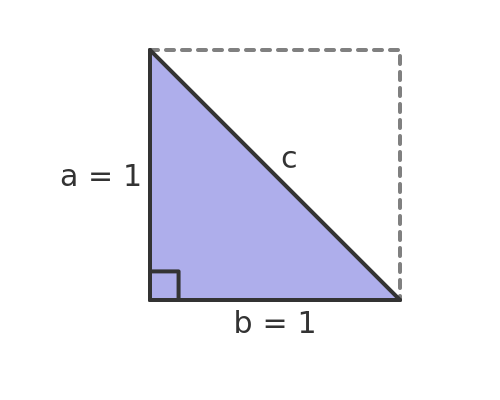The interesting thing about this triangle is that it is half of a square. The dashed grey line shows how we can add a second, identical triangle to make a square. The square has an area of 1 because its side is length 1.

We can find the length of the side c by setting a and b to 1 in the original equation: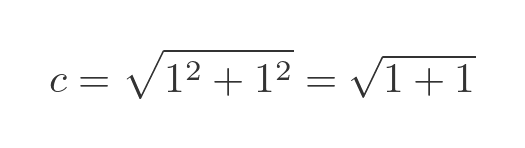This gives: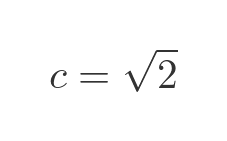So this time the hypotenuse isn't an integer value, it is the square root of 2.

We can see why this might be the case in this diagram: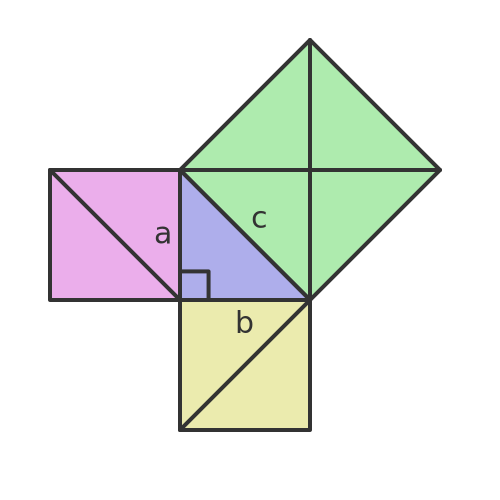The two small squares are each made up of two triangles that are congruent with the original triangle. This means that they are identical in size and shape.

The big square is made up of four triangles that are also congruent with the original triangle.

Even without using Pythagoras' theorem, we can see that the area of the big square is equal to the sum of the areas of the two small squares.

Since each small square has an area of 1, the big square has an area of 2. And since the area is equal to the square of the side length, this tells us that the big square has a side length of root 2.

## Applications of Pythagoras' theorem

We can use Pythagoras' theorem to solve a right-angled triangle. If we know any two sides we can find the third side.

This can be useful in geometry problems, but it also has other applications. For example:

• We can find the length of the diagonal of a rectangle. This can tell us what the longest item we can fit in a box of a particular size, for example.
• We can find the shortest distance between two points on a graph.
• We can find the length of a ladder we would need to reach a window.